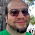## Saturday, May 30, 2015

### HP Prime and TI-84 Plus: Runge Kutta 4th Order

HP Prime and TI-84 Plus:  Runge Kutta 4th Order

The Runge Kutta 4th Order is a method for solving differential equations involving the form:  dy/dx = f(x,y), where:

x_n+1 = x_n + h
y_n+1 = y_n + (k1 + 2*k2 + 2*k3 + k4)/6

Where:

k1 = h * f(x_n, y_n)
k2 = h * f(x_n + h/2, y_n + k1/2)
k3 = h * f(x_n + h/2, y_n + k2/2)
k4 = h * f(x_n + h, y_n + k3)

Variables used:

A = x_n
B = y_n
C = x_n+1
D = y_n+1
H = step
K = k1
L = k2
M = k3
N = k4

Results are stored in lists L1 and L2.  L1 represents the x coordinates, L2 represents the y coordinates.

This adopts the RK4 method that I programmed for the Casio fx-5800p, which you can see here:  http://edspi31415.blogspot.com/2015/01/fx-5800p-runge-kutta-4th-order-method.html

The screen shots show the example dy/dx = x^2 + y^2/3, with initial conditions y(0) = 1.  10 steps are plotted with step size 0.1.

HP Prime:  DIFFTBL and RK4

Both DIFFTBL and RK4 return the same output.  The difference is that DIFFTBL uses an Input box and no-pass through arguments and RK4 uses five pass-through arguments.  This way, RK4 could be used as a subroutine.

Both return the results in a matrix, M1.  Rev. 7820 firmware is used.

DIFFTBL:
EXPORT DIFFTBL()
BEGIN
// EWS 2015-05-29
HAngle:=0;
// Input
LOCAL f;

INPUT({{f,},A,B,H,S},"Data Input",
{"dy/dx=","x0=","y0=","Step=",
"# Steps="});
L1:={A}; L2:={B};
// Main Loop
FOR I FROM 1 TO S DO
// k1
X:=A;
Y:=B;
K:=H*EVAL(f);
// k2
X:=A+H/2;
Y:=B+K/2;
L:=H*EVAL(f);
// k3
Y:=B+L/2;
M:=H*EVAL(f);
// k4
X:=A+H;
Y:=B+M;
N:=H*EVAL(f);
// point
A:=A+H;
B:=B+(K+2*L+2*M+N)/6;
L1:=CONCAT(L1,{A});
L2:=CONCAT(L2,{B});
END;
M1:=list2mat(CONCAT(L1,L2),
SIZE(L1));
M1:=TRN(M1);
RETURN M1;

END;

RK4:
EXPORT RK4(f,A,B,H,S)
BEGIN
// EWS 2015-05-29
HAngle:=0;
// Input

L1:={A}; L2:={B};
// Main Loop
FOR I FROM 1 TO S DO
// k1
X:=A;
Y:=B;
K:=H*EVAL(f);
// k2
X:=A+H/2;
Y:=B+K/2;
L:=H*EVAL(f);
// k3
Y:=B+L/2;
M:=H*EVAL(f);
// k4
X:=A+H;
Y:=B+M;
N:=H*EVAL(f);
// point
A:=A+H;
B:=B+(K+2*L+2*M+N)/6;
L1:=CONCAT(L1,{A});
L2:=CONCAT(L2,{B});
END;
M1:=list2mat(CONCAT(L1,L2),
SIZE(L1));
M1:=TRN(M1);
RETURN M1;

END;

TI-84 Plus:  DIFFPLOT

DIFFPLOT plots the results in a statistics xy-plot.  The equation variable Y0 is used, but subsequently deleted.  I left out any color commands to make this easily adoptable for both the monochrome and color TI-84 Plus calculators.

Do not forget to enclose dy/dx in quotes, unless an input error occurs

Disp "DIFF. EQUATION"
Disp "Y₀=DY/DX"
Disp "USE QUOTES"
Input Y₀
Input "X0=",A
Input "Y0=",B
Input "STEP=",H
Input "NO. STEPS=",S
{A}→L₁
{B}→L₂
For(I,1,S)
A→X:B→Y
H*Y₀→K
A+H/2→X:B+K/2→Y
H*Y₀→L
B+L/2→Y
H*Y₀→M
A+H→X:B+M→Y
H*Y₀→N
A+H→A
B+(K+2*L+2*M+N)/6→B
augment(L₁,{A})→L₁
augment(L₂,{B})→L₂
End
FnOff 0
PlotsOn 1
Plot1(xyLine,L₁,L₂)
ZoomStat

This blog is property of Edward Shore.  2015.

1.very good do you make the rules of simpson's and trapezoidal?

Thanks

Me email: ezequielgontijo@gmail.com

1.Ezequiel,

I usually find the integral functions to be adequate, but this is a very good suggestion for a future topic. Thanks,

Eddie

2.Ezequiel,

For the TI-84 Plus CE and HP Prime:
Trapezoidal Rule:
http://edspi31415.blogspot.com/2016/06/hp-prime-and-ti-84-plus-ce-trapezoidal.html
Simpsons Rule:
http://edspi31415.blogspot.com/2016/06/hp-prime-and-ti-84-plus-ce-simpsons-rule.html

2.Hi! Could you please make a video on how I get the RK4 running ? I am having trouble with it.
Thank you!

1.Please Edward, I don't know how to install your app on my HP Prime. I'm having some problems too.
Thank you

### Retro Review: Radio Shack EC-2024

Retro Review:  Radio Shack EC-2024 Quick Facts Company:  Radio Shack Years in Production: Around 1994 Original Price: \$24.99 Bat...